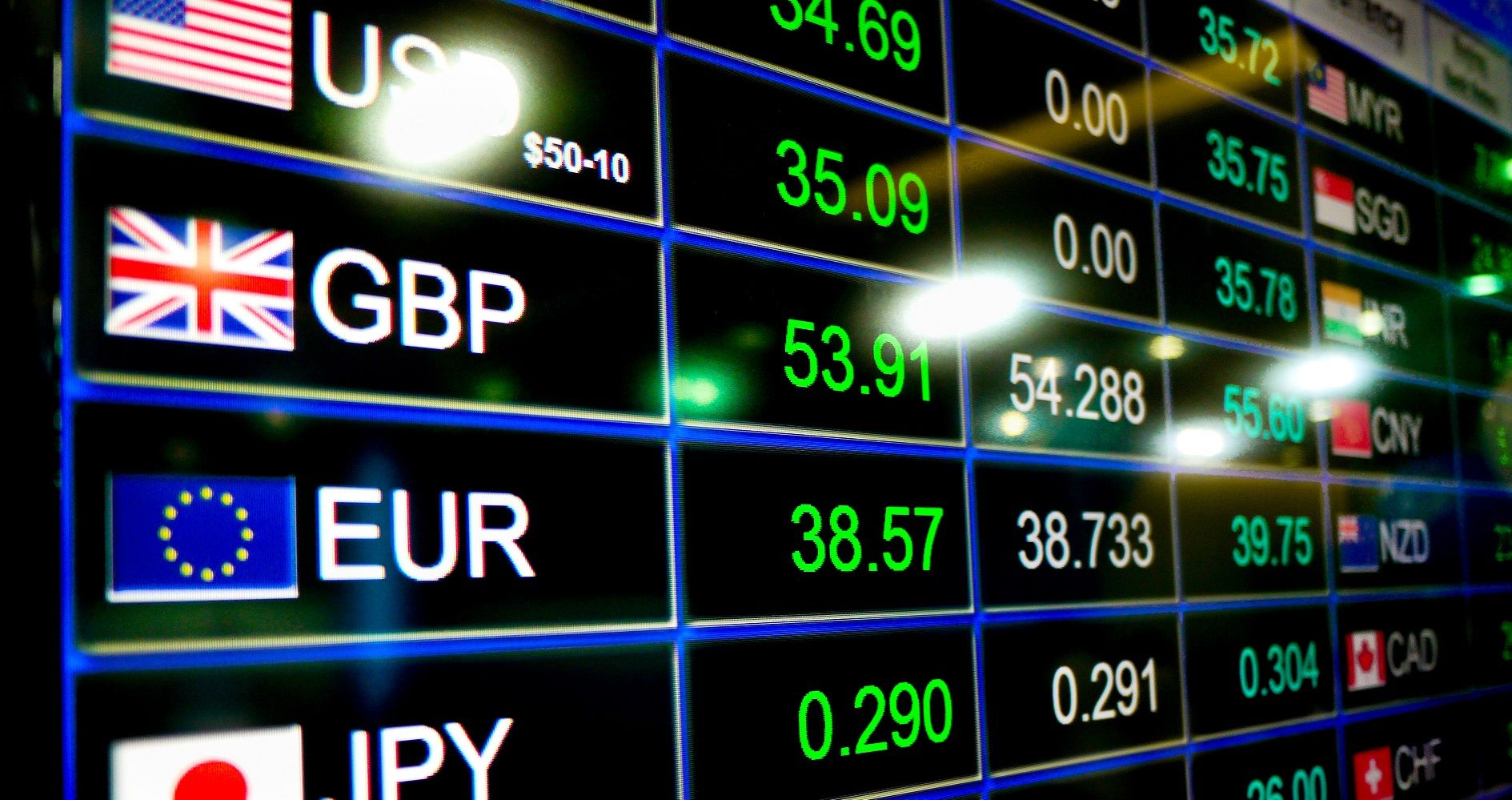# International Fisher Effect (IFE)

The difference between the nominal interest in two countries is directly proportional to the changes in their currencies

## What is the International Fisher Effect (IFE)?

The International Fisher Effect (IFE) states that the difference between the nominal interest rates in two countries is directly proportional to the changes in the exchange rate of their currencies at any given time. Irving Fisher, a U.S. economist,  developed the theory.The International Fisher Effect is based on current and future nominal interest rates, and it is used to predict spot and future currency movements. The IFE is in contrast to other methods that use pure inflation to try to predict and understand movements in the exchange rate.

### How the International Fisher Effect was Conceptualized

The International Fisher Effect theory was recognized on the basis that interest rates are independent of other monetary variables and that they provide a strong indication of how the currency of a specific country is performing. According to Fisher, changes in inflation do not impact real interest rates, since the real interest rate is simply the nominal rate minus inflation.

The theory assumes that a country with lower interest rates will see lower levels of inflation, which will translate to an increase in the real value of the country’s currency in comparison to another country’s currency. When interest rates are high, there will be higher levels of inflation, which will result in the depreciation of the country’s currency.

### How to Calculate the Fisher Effect

The formula for calculating the IFE is as follows:

E = [(i1-i2) / (1+ i2)]  ͌  (i1-i2)

Where:

• E = Percentage change in the exchange rate of the country’s currency
• I= Country’s A’s Interest rate
• I= Country’s B’s Interest rate

#### Example

Let’s take the example of two currencies, the USD (the United States) and the CAD (the Canadian Dollar). The USD/CAD spot exchange rate is 1.30, and the interest rate of the United States is 5.0% while that of Canada is 6.0%.

Based on the IFE assumption, the country with a higher interest rate, Canada in this case, will see a higher inflation rate and will experience a depreciation of its currency. The future spot rate is calculated by taking the spot rate and multiplying it by the ratio of the foreign interest rate to the domestic interest rate, as shown below:

1.3 x (1.05/1.06) = 1.312

Given the future spot rate, the International Fisher Effect assumes that the CAD currency will depreciate against the USD. 1 USD will exchange into 1.312 CAD, up from the original rate of 1.30. On one hand, investors will receive a lower interest rate on the USD currency, but on the other hand, they will gain from an increase in the value of the US currency.

For the IFE to work, several assumptions must be made. Some of the assumptions include the free flow of capital between countries, the integration of capital markets, and the lack of control on the currency for trade purposes.

### The Relevance of the International Fisher Effect

In the short term, the International Fisher Effect is seen as an unreliable variable of estimating the price movements of a currency, due to the existence of other factors that affect exchange rates. The factors also exert an effect on the prediction of nominal interest rates and inflation.

However, in the long run, the IFE is viewed as a more reliable variable to determine the effect of changes in nominal interest rates on shifts in exchange rates.

### Fisher Effect vs. International Fisher Effect

The Fisher effect describes the relationship between interest rates and the rate of inflation. It proposes that the nominal interest rate in a country is equal to the real interest rate plus the inflation rate, which means that the real interest rate is equal to the nominal rate of interest minus the rate of inflation.

Therefore, any increase in the rate of inflation will result in a proportional increase in the nominal interest rate, where the real interest rate is constant. For example, assume that the real interest rate is 5.5% and the rate of inflation changes from 2.5% to 3.5%. The nominal interest rate is calculated as follows:

(1 + Nominal Interest Rate) = (1+Real Interest Rate) (1+Inflation Rate)

Nominal = (1+0.055) (1+0.025) – 1

= (1.055) (1.025) – 1

= 0.081 or 8.1%

Nominal = (1.055) (1.035) – 1

= 0.092 or 9.2%

Therefore, the nominal interest rate would’ve increased from 8.1% when the inflation rate was 2.5% to 9.2% when the rate of inflation increases to 3.5%.

The International Fisher expands on the Fisher Effect theory by suggesting that the estimated appreciation or depreciation of two countries’ currencies is proportional to the difference in their nominal interest rates. For example, if the nominal interest rate in the United States is greater than that of the United Kingdom, the former’s currency value should fall by the interest rate differential.

### More Resources

CFI is the official provider of the Financial Modeling and Valuation Analyst (FMVA)™ certification program, designed to transform anyone into a world-class financial analyst.

To keep learning and developing your knowledge of financial analysis, we highly recommend the additional CFI resources below:

• Effective Annual Interest Rate
• EUR/USD Currency Cross
• Economic Exposure